Courses

# Thermodynamics Potential Physics Notes | EduRev

## Physics : Thermodynamics Potential Physics Notes | EduRev

The document Thermodynamics Potential Physics Notes | EduRev is a part of the Physics Course Basic Physics for IIT JAM.
All you need of Physics at this link: Physics

Thermodynamic potentials are state functions that, together with the corresponding equations of state, describe the equilibrium behavior of a system as a function of so-called ”natural variables”. The natural variables are a set of appropriate variables that allow to compute other state functions by partial differentiation of the thermodynamic potentials.

Internal energy U
The basic relation of thermodynamics is given by the equation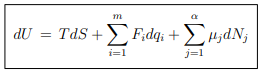...(5.1)
where {F, q} denote the set of conjugate intensive and extensive variables that characterize a system. For instance, for a gas
{F, q} → {−P, V },
and for a magnetic system
{F, q} → {B,M} .

Chemical potential. The number of particles in the system is a natural extensive
variable for the free energy, we did keep it hitherto constant. The number of particle of a distinct types j is denoted by Nj in (5.1), where j = 1, . . . , α. The respective intensive variable, µ (and respectively the µj ), is denoted the chemical potential.
The chemical potential become identical to the Fermi energy for a gas of Fermions (at low temperatures, as we will discuss in Sect. 13.3). Note that diffusion processes (e.g. across a membrane) may change individual Nj . The number of particles needs therefore not to be constant.

Internal energy of a gas. Eq. (5.1) is equivalent to
dU = T dS − P dV + µdN ,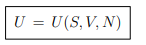...(5.2)
for a gas with one species of particle, which implies that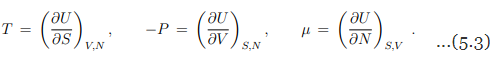Response functions. The experimentally important response functions are obtained by second-order differentiation of the internal energy,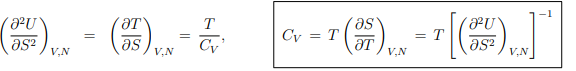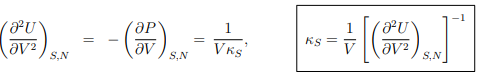Maxwell relations. A Maxwell relation follows, as discussed already in Sect. 4.4.2, from the differentiability of thermodynamic potentials. An example of a Maxwell relation derived from the differential of the internal energy U = U(S, V, N) is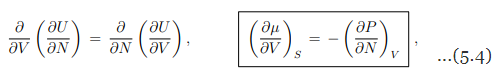which relates the change of the chemical potential µ with the volume V to the (negative of the) change of the pressure P with the number of particles N.

Monoatomic ideal gas
For the monoatomic ideal we did find hitherto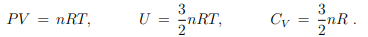We now use these relations to derive an expression for U in terms of its natural variables.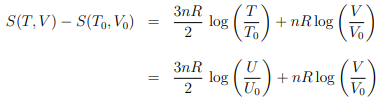of the ideal gas, as derived in Sect. 4.4.1, where we have used the ideal gas relation U = 3nRT/2 to substitute T and T0 with U and U0 in the second step.
With CV = 3nR/2 we may write equivalently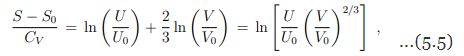which can be solved for the internal energy as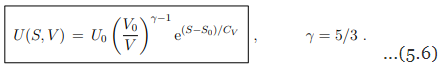Eq. (5.6) is the fundamental equation for the ideal gas, with U(S, V ) as the thermodynamic potential. S, V are the independent natural variables.

Thermodynamic potential vs. equation of state
The natural variables for U are S and V , which means that if the function U(S, V ) is known for a given system we can obtain –all– thermodynamic properties of the system through the differentiation of U(S, V ). The equation of state
U = U(T, V, N)  ...(5.7)
for the internal energy U is on the contrary –not– a thermodynamic potential. This is because the first derivatives of (5.7) yield the specific heat CV and the energy equation (4.12),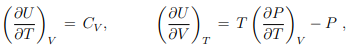but not the dependent variables S and P. Note the marked difference to (5.3).

Classical mechanics vs. thermodynamics
There is a certain analogy between the potential V and internal energy U of classical mechanics and thermodynamics respectively: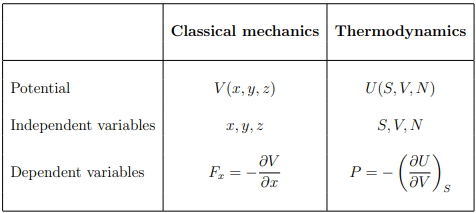Legendre transformation
A disadvantage of using U(S, V, N) as a thermodynamic potential is that the natural variable S is difficult to control in the lab. For practical purposes, it is more convenient to deal with other thermodynamic potentials that can be defined by making use of the

Legendre transformation.
Legendre transformations in classical mechanics. We recall the Legendre transformation connecting the Legendre function L(q, q˙) with the Hamilton function H(q, p), where q is the generalized coordinated and, q˙ and p respectively the velocity and the momentum, with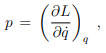at constant coordinate q, and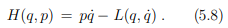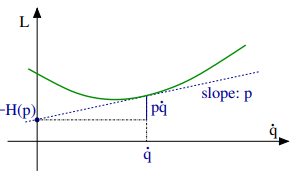A Legendre transformation is hence a variable transformation, q˙ → p, where one of the variables, here the momentum p, is defined by the slope of original function, viz by the slope of the Lagrange function L(p, q˙).

Legendre transformations in thermodynamics
As an example we consider the transformation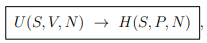from the internal energy U(S, V, N) to the enthalpy H(S, P, V ). The form is, as discussed shortly in Sect. 3.6,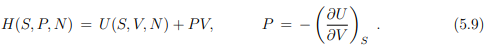Note that the pressure is defined as the (negative) slope of the internal energy U with respect to the volume V , in analogy to the transformation from the velocity q˙ to the momentum p = ∂L/∂q˙ in classical mechanics. Note however the different sign conventions between (5.9) and (5.8).

Differential. The Legendre transformation (5.9) allows to evaluate with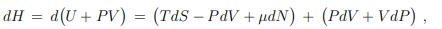the differential dH of the enthalpy in terms of its natural variables S, P and N. The result is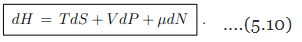The four basic thermodynamic potentials
A thermodynamic potential for a system with variable number of particles should depend on {µ, N} as well as a thermal variable and a mechanical variable, which can be (for a gas):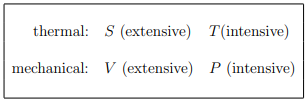The four possible combinations of these variables,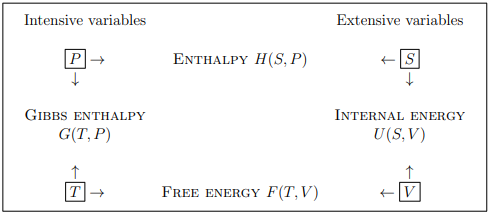define four basic thermodynamic potentials:
U(S, V, N) – internal energy
H(S, P, N) – enthalpy
G(T, P, N) – Gibbs enthalpy
F(T, V, N) – free energy
Gibbs enthalpy. We did already discuss the differentials (5.2), dU = T dS−P dV +µdN, and (5.10) of respectively the free energy U(S, V, N) and of the enthalpy H(S, P, N). For the Gibbs enthalpy one has in analogy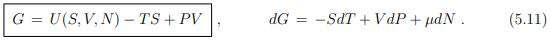Free energy. The natural variables T, V and N of the free energy F = F(T, V, N) are straightforward to access experimentally. The free energy is hence an often considered thermodynamic potential. It obeys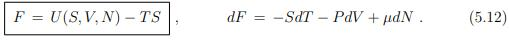Differential relation between free and internal energy. The Legendre transformation (5.12) between U and F allow to derive a differential relation between the free and internal energy,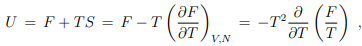which can be written in terms of the inverse temperature β = 1/(kBT) as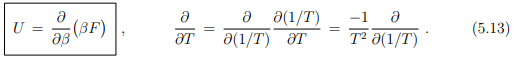It is generally easier to obtain explicit expressions for the internal energy U than for the free energy F. Above relation will be for this reason fundamental to the formulation of statistical mechanics, as discussed lateron in Sect. 9.2, as it allows to identify F.

Maxwell relations
The differentiability of the thermodynamic potentials leads to various Maxwell relations, such as the one given by Eq. (5.4). There are furthermore four Maxwell relations relating T, respectively S to P respectively V . We drop in the following the dependence on the number of particles N.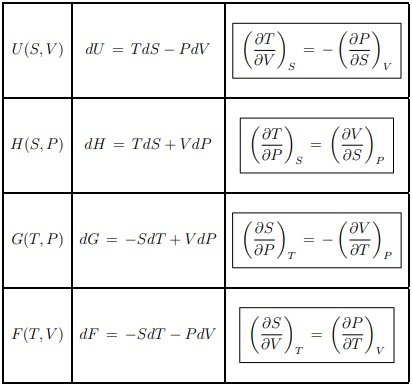Entropy
The differential of the entropy S = S(U, V, N) is obtained rewriting (5.2) as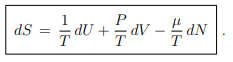...(5.14)
The conjugate (natural) intensive variables are here 1/T and P/T with respect to dU and dV respectively. It is hence not customary to perform Legendre transformations with respect to these variables.

Homogeneity relations
The thermodynamic potentials vary as extensive state properties if the volume and number of particles vary by a factor λ, i.e.,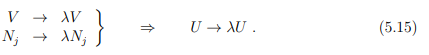Note that long-range interactions between particles, such as the unscreened Coulomb interaction, may invalidate (5.15). Surface effects will contribute in addition for the case of a mesoscopic system.
Extensive and intensive variables. Temperature is intensive, but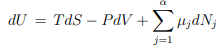extensive. It follows likewise that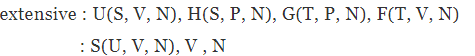intensive : T, P, µ
Scaling. Doubling the volume V will also double the free energy F(T, V, N). All extensive functions, viz all thermodynamic potentials obey hence the scaling relations of the sort of
F(T, λV, λN) = λF(T, V, N)
G(T, P, λN) = λG(T, P, N)    ...(5.16)
These functional dependencies express the homogeneity of the equilibrium thermodynamic state.
Gibbs-Duhem relation. Scaling relations are generically of fundamental importance in physics. Here we use the extensivity of G and differentiate (5.16) with respect to λ (at λ = 1),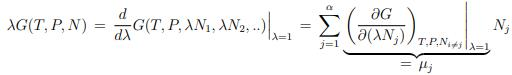We obtain then with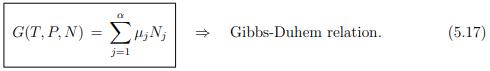the Gibbs-Duhem relation.

Chemical potential. When there is only one class of particles (α = 1),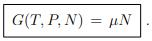...(5.18)
The chemical potential may hence be interpreted as Gibbs enthalpy per particle.

Representation of the internal energy. The Gibbs-Duhem relation (5.18) allows to rewrite the other thermodynamic potential. We recall, e.g. the Legendre transform (5.11),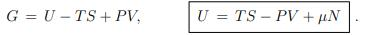Note, however, that this representation is only seemingly simple.

Grand canonical potential
In a system that interchanges particles with a reservoir, the chemical potential is constant and the number of particles changes accordingly. In order to describe such a system, we introduce the grand canonical potential Ω(T, V, µ) as the Legendre transform of F(T, V, N), where the natural variable N is replaced by µ,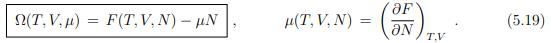The total differential of Ω is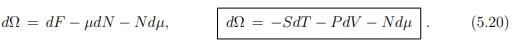Through differentiation of Ω we can calculate S, P and N as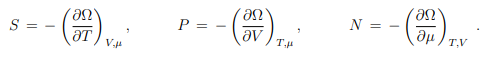Thermal equation of state. From the Gibbs Duhem relation (5.18), G = µN, it follows that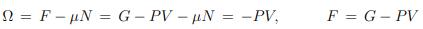and hence that the thermal equation of state P = P(T, V ), see Eq. (4.11), takes the from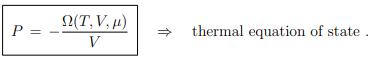Differential relation betwen Ω and U. With, (5.12), F = U − T S, we may write (5.19) as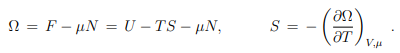Reanranging the terms then leads to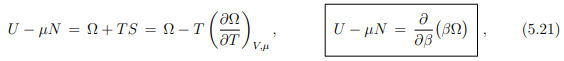where we have used in analogy to (5.13) that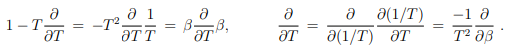Equilibrium conditions
Our discussion of thermodynamic potentials dealt hitherto mostly with the equilibrium state. The second law of thermodynamics,
T dS ≥ δQ = dU + P dV − µdN ,     ...(5.22)
allows however also to study the impact of irreversible processes, that is the evolution of the system toward the equilibrium. We have used for (5.22) the differential form of (4.6) together with the generalized first law
dU = δQ + δW + µdN,     δW = −P dV ,
where we have taken the δW appropriate for a gas.

Equilibrium internal energy. The entropy is an exact differential dS = δQ/T at equilibrium. One can then consider situations like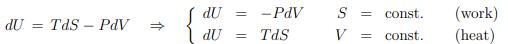where selected state variables are constant. We will now generalize this discussion.

Principle of maximal entropy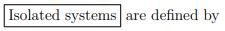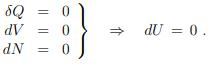The second law (5.22) tells us then that
ΔS ≥ 0
dS = 0 in equilibrium .

Principle of maximal entropy.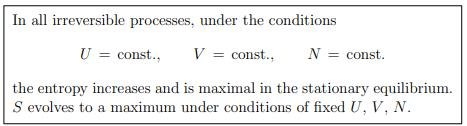Two isolated system. Consider two isolated systems 1 and 2 separated by a wall, which is however movable and which allows for the exchange of energy and particles. The variables V1, V2, U1, and U2, N1, N2 must obey the following constraints:
U = U1 + U2 = const. ⇒ dU1 = −dU2
V = V1 + V2 = const. ⇒ dV1 = −dV2
N = N1 + N2 = const. ⇒ dN1 = −dN2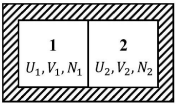The total entropy of 1 and 2 is likewise a sum of the individual entropies:
S = S(U1, V1, N1) + S(U2, V2, N2) = S1 + S2 .
Equilibrium conditions. Both systems will interchange particles and energy until equilibrium is attained. In the equilibrium state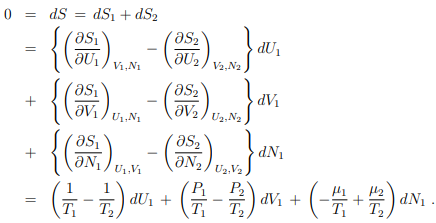where we have used the steady-state version of the second law (5.22), dS = dU + P dV − µdN.
Homogeneity condition. U1, V1, and N1 are independent variables. The prefactors of
dU1, dV1, and dN1 need hence to vanish individually:
T1 = T2 = T; P1 = P2 = P; µ1 = µ2 = µ .
Instead of two systems we can subdivide a given system in an arbitrary number of ways.
The system needs consequently to be homogeneous: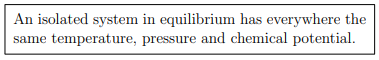Principle of minimal free energy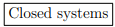in a reservoir with heat, but without work interchange, are defined by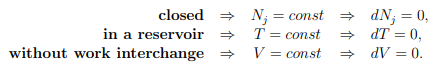The second law of thermodynamics (5.22) reduces then to
T dS ≥ dU + P dV − µdN = dU,     dV = 0 = dN .

Principle of minimal free energy. For constant temperature one has T dS = d(T S) and consequently that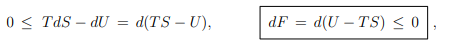where we have used (5.12), namely that F = U − T S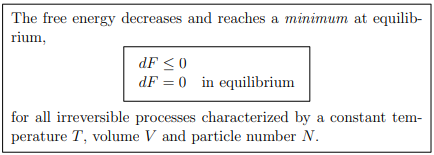Composite system. Consider a composite system in a heat reservoir at temperature T, whose particles can pass through the wall. It obeys the conditions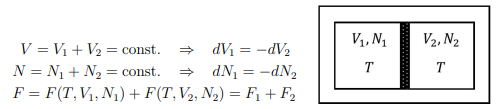In equilibrium,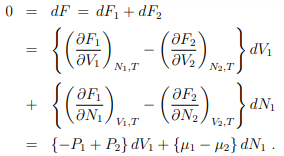With V1 and N1 being independent variables if follows that
P1 = P2 = P, µ1 = µ2 = µ .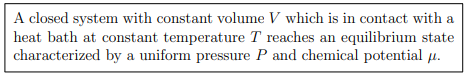Principle of minimal Gibbs energy. Generalizing above considerations we consider closed system in a heat reservoir, but at constant pressure P (previously V was constant). One can easily show that
dT = 0, dNj = 0, dP = 0
implies that
dG ≤ 0
dG = 0 in equilibrium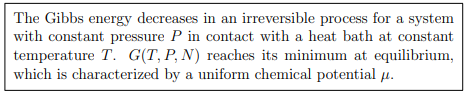Stationarity principles in information theory
From the microscopic point of view the equilibrium thermodynamic state is stationary in the sense that the positions and the velocities of the constituent particle change continuously. The ongoing reconfiguration of the microscopic state leaves however the macroscopic properties of the state intact.
Stationary probability distribution functions. A probability distribution functions p(y) quantifies the probability that the state y (an event) occurs, with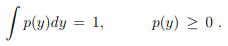It is the task of statistical mechanics to find the expression for p(y). Statistical mechanics dealing hence with probability distribution functions which are stationary with respect to the reconfiguration of microscopic states.

Entropy and disorder.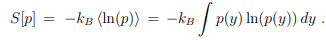It measures the amount of disorder in the sense that a flat (maximally disordered) distribution function p(y) has the largest entropy S[p]. Here we have written S = S[p] as a functional of p = p(y). This connection allows us to reinterpret the principle of maximal entropy derived in Sect. 5.5.1.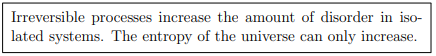Entropy and information. Information theory deals with information processing as described by probability distribution functions. The central quantity of information theory is the Shannon entropy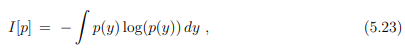which is identical, apart from an overall factor, kB, to the thermodynamic entropy. The Shannon entropy is however used as a measure of the information content. The context is here information transmitted via communication channels.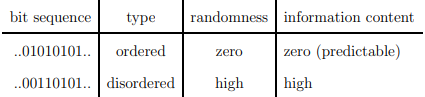You do not learn anything new if you can predict what another person says.

Shannon’s source coding theorem. A precise connections between the information theoretical entropy I[p] and the information content of a communication channel is provided by the Shannon coding theorem, which states the I[p] provides a lower bound for lossless compression. Information is inevitably lost when compressing more.

Life as a stationary state. Life is by definition a stationary state of matter which retains its functionality despite the continuously ongoing turnover of its constituent elements. The proteins, enzymes and cells of our body have lifetimes ranging typically from hours to months.

Equilibrium principles in the neurosciences. The stationary equilibrium of the brain with both the environment and the body is dependent on a continuous flux of information. Information theoretical principles are consequently of widespread use in the neurosciences. An example is the free energy principle, as proposed by Carl Friston. It suggests that the brain may be minimizing the information-theoretical analog of the thermodynamic free energy.

Summary of thermodynamic potentials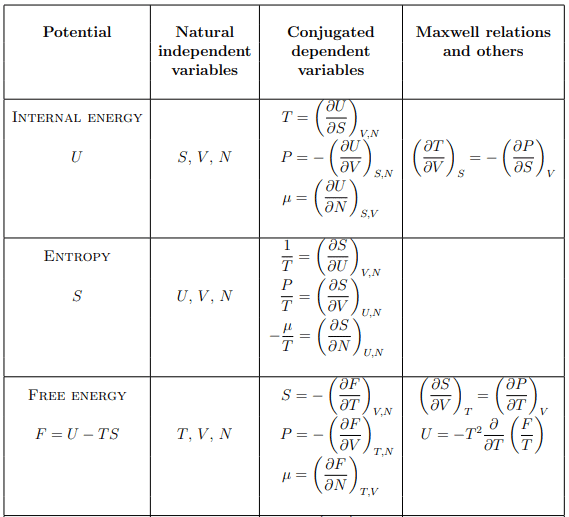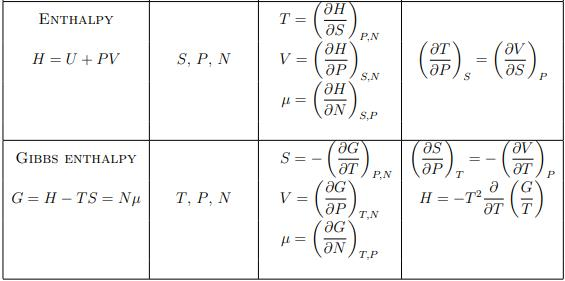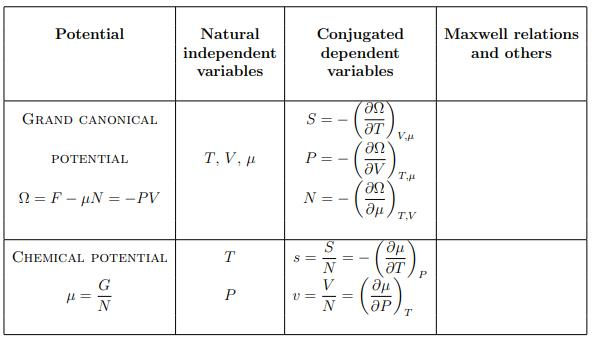Offer running on EduRev: Apply code STAYHOME200 to get INR 200 off on our premium plan EduRev Infinity!

,

,

,

,

,

,

,

,

,

,

,

,

,

,

,

,

,

,

,

,

,

;